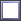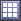# Reliability Plot Post Processing

Visualize representations of system reliability.

## Visualize Representation of System Reliability

Visualize the representation of system reliability in the Reliability Plot post processing tab.

1. From the Post Processing step, click the Reliability Plot tab.
2. Using the Channel selector, select the channel(s) to plot.
3. Analyze the plot.

The relationship between the desired threshold and the reliability of the system is plotted.

Refer to the vertical axis to locate a desired reliability and find the corresponding x-axis value of the curve to identify the required threshold. For example, "To have 95% reliability I would have to design this value." You can also find a threshold from the x-axis and locate the corresponding reliability. For example, "91% of my designs are below X value". The latter use case is more common.
Tip: Display selected data in a single plot or separate plots by switching the Multiplot option between(single plot) and(multiple plots).